# 平衡二叉树（AVL树）的基本操作

0x00、平衡二叉树的定义

平衡二叉树（AVL树）是一种特殊的二叉搜索树，只是在二叉搜索树上增加了对"平衡"的需求。

假如一棵二叉搜索树，按照“1,2,3,4,5”的顺序插入数据，会发现二叉树甚至变成了一个线性的链表状结构，这样查找数据的时间复杂度就会达到O(n)，为了优化这一点，使二叉查找树时间复杂度保持O(log n)的级别，我们就需要调整树的插入方式，使之保持住树的结构。

这里引入一个定义：

平衡因子：二叉树的某个节点的左子树与右子树高度之差称为结点的平衡因子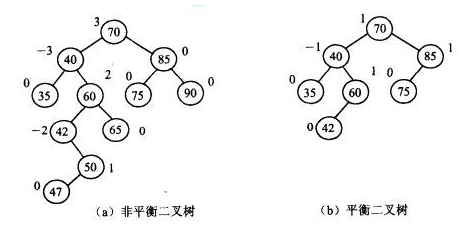0x01、平衡二叉树的C语言实现

``````struct node{
int data;    //数据域
int height;  //当前子树高度
node* lchild, *rchild;        //左右孩子
};``````

``````int getHeight(node* root){
return root == NULL ? 0 : root->height;
}``````

``````int getBalanceFactor(node* root){
return getHeight(root->lchild) - getHeight(root->rchild);
}``````

``````void updateHeight(node* root){
root->height = max(getHeight(root->lchild), getHeight(root->rchild)) + 1;
}``````

0x02、平衡二叉树的查找

AVL树的查找与一般二叉搜索树的查找方法一样。代码如下：

``````void search(node* root, int data){
if(!root) return;    //查找失败
if(root->data == data)//找到了
printf("%d", root->data);
if(root->data > data) //大了，访问左节点
search(root->lchild, data);
else search(root->rchild, data);
}``````

0x03、平衡二叉树的插入操作

平衡二叉树的插入操作较为复杂，我们为了保持二叉树的平衡状态，就要在二叉树失衡的时候对二叉树进行旋转。

常见的旋转方式有LL型（单次右旋）、RR型（单次左旋）、LR型（先左旋，再右旋）、RL型（先右旋，再左旋）

下面我们进行详细介绍：

1、LL型（单次右旋）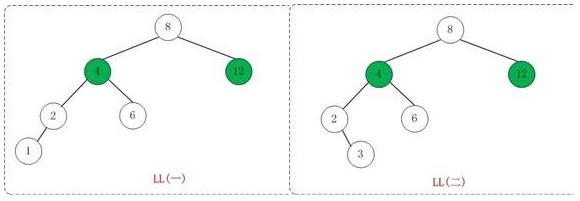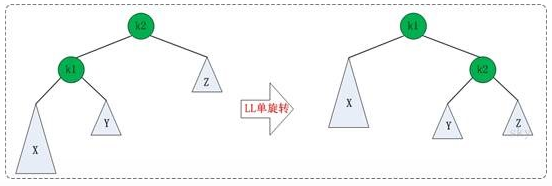``````void LLrotation(node* &root){
node* temp = root->lchild;    //让temp=K1
root->lchild = temp->rchild;    //让root的左节点变为Y
temp->rchild = root;    //让新根K1的右节点指向K2
//以上，旋转完毕
updateHeight(temp);    //更新新根的节点高度
updateHeight(root);    //更新老根的节点高度
root = temp;    //把树的根换成我们定义的新根
}``````

2、RR型（单次左旋）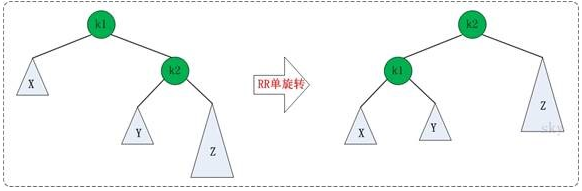C语言实现：

``````void RRrotation(node* &root){
node* temp = root->rchild;    //另temp=K2
root->rchild = temp->lchild;    //旧根的右节点为新根的左节点
temp->lchild = root;    //新根的右节点等于旧根
//以上，旋转完毕
updateHeight(temp);    //更新节点高度
updateHeight(root);
root = temp;    //重赋值
}``````

3、LR型（先单次左旋，后单次右旋）4的高度为3； 而12的高度为1，平衡因子即高度差为2，这是由于根节点的左子树的右子树造成了平衡因子为2的失衡，通常采用LR型旋转，即先左旋，后右旋。C语言实现：

``````void LRrotation(node* &root){
RRrotation(root->lchild);    //先对根节点的左孩子单次左旋
LLrotation(root);
}``````

4、RL型（先单次右旋，后单次左旋）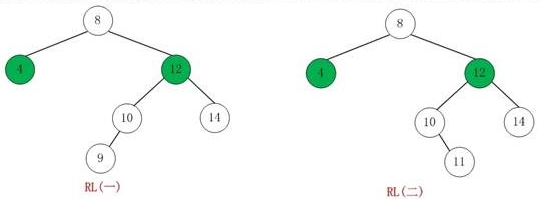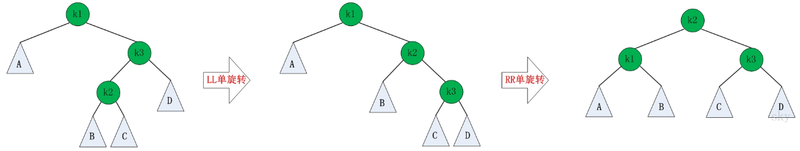C语言实现：

``````void RLrotation(node* &root){
LLrotation(root->rchild);    //先对根节点的左孩子单次左旋
RRrotation(root);
}``````

 树型 判定条件 调整方法 LL（单次右旋） BF(root) = 2；BF(root->lchild) = 1 对root单次右旋 RR（单次左旋） BF(root) =-2；BF(root->rchild) =-1 对root单次左旋 LR（先左后右） BF(root) = 2；BF(root->lchild) =-1 对root->lchild进行左旋，再对root进行右旋 RL（先右后左） BF(root) =-2；BF(root->rchild) = 1 对root->rchild进行右旋，再对root进行左旋

*BF代表平衡因子

0x04、二叉平衡树的插入

``````void insert(node* &root, int data){
if(root == NULL){
root = new node;
root->data = data;
root->lchild = root->rchild = NULL;
}
if(root->data <= data)
insert(root->rchild, data);
else insert(root->lchild, data);
}``````

C语言实现：

``````void insert(node* &root, int data){
if(root == NULL){    //插入位置
root = new node;
root->data = data;
root->lchild = root->rchild = NULL;
root->height = 1;    //由于插入的时候是叶子节点，所以初始高度为1
return;
}
if(root->data > data){    //节点数据大，往左插入
insert(root->lchild, data);    //继续递归寻找插入位置
updataHeight(root);        //因为插入了一个节点，所以树根的高度会发生变化
if(getBalanceFactor(root) == 2)    //根节点平衡因子为2
if(getBalanceFactor(root->lchild) == 1) //根节点左孩子平衡因子为1，根据表格，采用LL型
LLrotation(root);
else if(getBalanceFactor(root->lchild) == -1)   //根节点左孩子平衡因子为-1，LR型
LRrotation(root);
}
else{    //节点数据小，往右插入
insert(root->rchild, data);
updataHeight(root);
if(getBalanceFactor(root) == -2){
if(getBalanceFactor(root->rchild) == -1) //RR型
RRrotation(root)
else if(getBalanceFactor(root->rchild) == 1)//RL型
RLrotation(root);
}
}
}``````